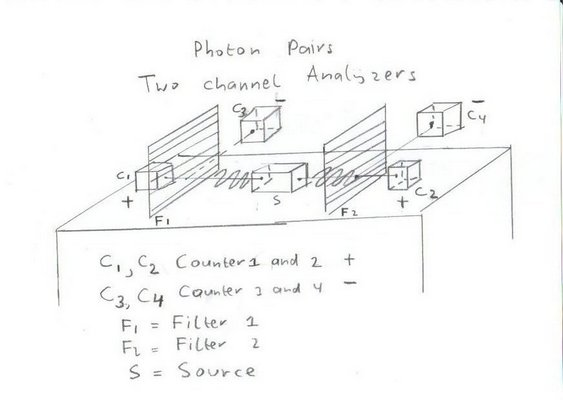## Experiment 3 with Entangled photonsThe assumption is:
• That the left photon moves towards the left and is polarised in the horizontal plane .
• That the right photon moves towards the right and is also polarised in the horizontal plane .
The idea behind this experiment is to observe and compare the sequence of events when you insert a two channel polariser in both the left and the right beam with the aid of a Coincidence Monitor.

The result of the test will be 2 sequence of events, showing the arrival times of the photons.

```Counter 1: x    x x    x       x     x        x    x  x       tn1 = 9
Counter 3:   x       x   x  x    x      x   x      x    x   x tn3 = 10
Counter 2: x    x      x       x          x x x    x  x       tn2 = 9
Counter 4:   x       x      x    x   x  x        x x    x   x tn4 = 10
```
The above pattern shows that in total 20 photon pairs are created, but that in four cases only one counter increments and in one case all the four counters.

Those type of errors should be included in any discussion about the results.
As such we get:

• 13 cases were either both counter 1 and counter 3 or counter 2 and counter 4 are incremented.
• 2 cases were either both counter 1 and counter 4 or counter 2 and counter 3 are incremented.
• 5 cases with only one counter or all the four counters incrementing
Meaning there is only 65% agreement with the prediction that both photons move in the same plane.
My expectation that with a real life experiment the agreement wil be higher, but not at 100%.